# Resources tagged with: Addition & subtraction

Filter by: Content type:
Age range:
Challenge level:

### There are 211 results### Football Sum

##### Age 11 to 14 Challenge Level:

Find the values of the nine letters in the sum: FOOT + BALL = GAME### Being Resilient - Primary Number

##### Age 5 to 11 Challenge Level:

Number problems at primary level that may require resilience.### Cayley

##### Age 11 to 14 Challenge Level:

The letters in the following addition sum represent the digits 1 ... 9. If A=3 and D=2, what number is represented by "CAYLEY"?##### Age 11 to 14 Challenge Level:

If you take a three by three square on a 1-10 addition square and multiply the diagonally opposite numbers together, what is the difference between these products. Why?### Digit Sum

##### Age 11 to 14 Challenge Level:

What is the sum of all the digits in all the integers from one to one million?##### Age 5 to 11 Challenge Level:

Try out this number trick. What happens with different starting numbers? What do you notice?### As Easy as 1,2,3

##### Age 11 to 14 Challenge Level:

When I type a sequence of letters my calculator gives the product of all the numbers in the corresponding memories. What numbers should I store so that when I type 'ONE' it returns 1, and when I type. . . .### Making Pathways

##### Age 7 to 11 Challenge Level:

Can you find different ways of creating paths using these paving slabs?### The Patent Solution

##### Age 11 to 14 Challenge Level:

A combination mechanism for a safe comprises thirty-two tumblers numbered from one to thirty-two in such a way that the numbers in each wheel total 132... Could you open the safe?### Eleven

##### Age 11 to 14 Challenge Level:

Replace each letter with a digit to make this addition correct.### Chameleons

##### Age 11 to 14 Challenge Level:

Whenever two chameleons of different colours meet they change colour to the third colour. Describe the shortest sequence of meetings in which all the chameleons change to green if you start with 12. . . .### Like Powers

##### Age 11 to 14 Challenge Level:

Investigate $1^n + 19^n + 20^n + 51^n + 57^n + 80^n + 82^n$ and $2^n + 12^n + 31^n + 40^n + 69^n + 71^n + 85^n$ for different values of n.### Oddly

##### Age 7 to 11 Challenge Level:

Find the sum of all three-digit numbers each of whose digits is odd.### Book Codes

##### Age 7 to 11 Challenge Level:

Look on the back of any modern book and you will find an ISBN code. Take this code and calculate this sum in the way shown. Can you see what the answers always have in common?### Napier's Bones

##### Age 7 to 11 Challenge Level:

The Scot, John Napier, invented these strips about 400 years ago to help calculate multiplication and division. Can you work out how to use Napier's bones to find the answer to these multiplications?### Kids

##### Age 11 to 14 Challenge Level:

Find the numbers in this sum### Aba

##### Age 11 to 14 Challenge Level:

In the following sum the letters A, B, C, D, E and F stand for six distinct digits. Find all the ways of replacing the letters with digits so that the arithmetic is correct.### Tis Unique

##### Age 11 to 14 Challenge Level:

This addition sum uses all ten digits 0, 1, 2...9 exactly once. Find the sum and show that the one you give is the only possibility.### Jugs of Wine

##### Age 11 to 14 Challenge Level:

You have four jugs of 9, 7, 4 and 2 litres capacity. The 9 litre jug is full of wine, the others are empty. Can you divide the wine into three equal quantities?### Arrange the Digits

##### Age 11 to 14 Challenge Level:

Can you arrange the digits 1,2,3,4,5,6,7,8,9 into three 3-digit numbers such that their total is close to 1500?### Largest Number

##### Age 11 to 14 Challenge Level:

What is the largest number you can make using the three digits 2, 3 and 4 in any way you like, using any operations you like? You can only use each digit once.### Always the Same

##### Age 11 to 14 Challenge Level:

Arrange the numbers 1 to 16 into a 4 by 4 array. Choose a number. Cross out the numbers on the same row and column. Repeat this process. Add up you four numbers. Why do they always add up to 34?### Score

##### Age 11 to 14 Challenge Level:

There are exactly 3 ways to add 4 odd numbers to get 10. Find all the ways of adding 8 odd numbers to get 20. To be sure of getting all the solutions you will need to be systematic. What about. . . .### Subtraction Surprise

##### Age 7 to 14 Challenge Level:

Try out some calculations. Are you surprised by the results?### Being Collaborative - Primary Number

##### Age 5 to 11 Challenge Level:

Number problems at primary level to work on with others.### Number Differences

##### Age 7 to 11 Challenge Level:

Place the numbers from 1 to 9 in the squares below so that the difference between joined squares is odd. How many different ways can you do this?### Take Three Numbers

##### Age 7 to 11 Challenge Level:

What happens when you add three numbers together? Will your answer be odd or even? How do you know?### Cows and Sheep

##### Age 7 to 11 Challenge Level:

Use your logical reasoning to work out how many cows and how many sheep there are in each field.### Escape from the Castle

##### Age 7 to 11 Challenge Level:

Skippy and Anna are locked in a room in a large castle. The key to that room, and all the other rooms, is a number. The numbers are locked away in a problem. Can you help them to get out?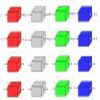### Machines

##### Age 7 to 11 Challenge Level:

What is happening at each box in these machines?### Making Sense of Positives and Negatives

##### Age 11 to 14

This article suggests some ways of making sense of calculations involving positive and negative numbers.### A Mixed-up Clock

##### Age 7 to 11 Challenge Level:

There is a clock-face where the numbers have become all mixed up. Can you find out where all the numbers have got to from these ten statements?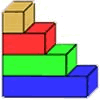### Rod Measures

##### Age 7 to 11 Challenge Level:

Using 3 rods of integer lengths, none longer than 10 units and not using any rod more than once, you can measure all the lengths in whole units from 1 to 10 units. How many ways can you do this?### Spell by Numbers

##### Age 7 to 11 Challenge Level:

Can you substitute numbers for the letters in these sums?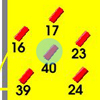### Throw a 100

##### Age 7 to 11 Challenge Level:

Can you score 100 by throwing rings on this board? Is there more than way to do it?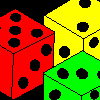##### Age 7 to 11 Challenge Level:

Three dice are placed in a row. Find a way to turn each one so that the three numbers on top of the dice total the same as the three numbers on the front of the dice. Can you find all the ways to do. . . .### Zios and Zepts

##### Age 7 to 11 Challenge Level:

On the planet Vuv there are two sorts of creatures. The Zios have 3 legs and the Zepts have 7 legs. The great planetary explorer Nico counted 52 legs. How many Zios and how many Zepts were there?### Sisters and Brothers

##### Age 7 to 11 Challenge Level:

Cassandra, David and Lachlan are brothers and sisters. They range in age between 1 year and 14 years. Can you figure out their exact ages from the clues?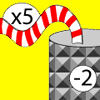### Function Machines

##### Age 7 to 11 Challenge Level:

If the numbers 5, 7 and 4 go into this function machine, what numbers will come out?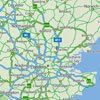### Journeys

##### Age 7 to 11 Challenge Level:

Investigate the different distances of these car journeys and find out how long they take.### Sometimes We Lose Things

##### Age 7 to 11 Challenge Level:

Well now, what would happen if we lost all the nines in our number system? Have a go at writing the numbers out in this way and have a look at the multiplications table.### Bundles of Cubes

##### Age 7 to 11 Challenge Level:

Watch this animation. What do you notice? What happens when you try more or fewer cubes in a bundle?### The Clockmaker's Birthday Cake

##### Age 7 to 11 Challenge Level:

The clockmaker's wife cut up his birthday cake to look like a clock face. Can you work out who received each piece?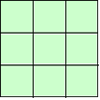### 1, 2, 3 Magic Square

##### Age 7 to 11 Challenge Level:

Arrange three 1s, three 2s and three 3s in this square so that every row, column and diagonal adds to the same total.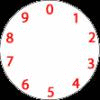### Dividing a Cake

##### Age 7 to 11 Challenge Level:

Annie cut this numbered cake into 3 pieces with 3 cuts so that the numbers on each piece added to the same total. Where were the cuts and what fraction of the whole cake was each piece?### The Deca Tree

##### Age 7 to 11 Challenge Level:

Find out what a Deca Tree is and then work out how many leaves there will be after the woodcutter has cut off a trunk, a branch, a twig and a leaf.### A-magical Number Maze

##### Age 7 to 11 Challenge Level:

This magic square has operations written in it, to make it into a maze. Start wherever you like, go through every cell and go out a total of 15!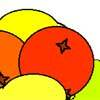### Oranges and Lemons

##### Age 7 to 11 Challenge Level:

On the table there is a pile of oranges and lemons that weighs exactly one kilogram. Using the information, can you work out how many lemons there are?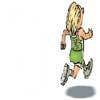### Rocco's Race

##### Age 7 to 11 Short Challenge Level:

Rocco ran in a 200 m race for his class. Use the information to find out how many runners there were in the race and what Rocco's finishing position was.### Pyramids

##### Age 11 to 14 Challenge Level:

What are the missing numbers in the pyramids?